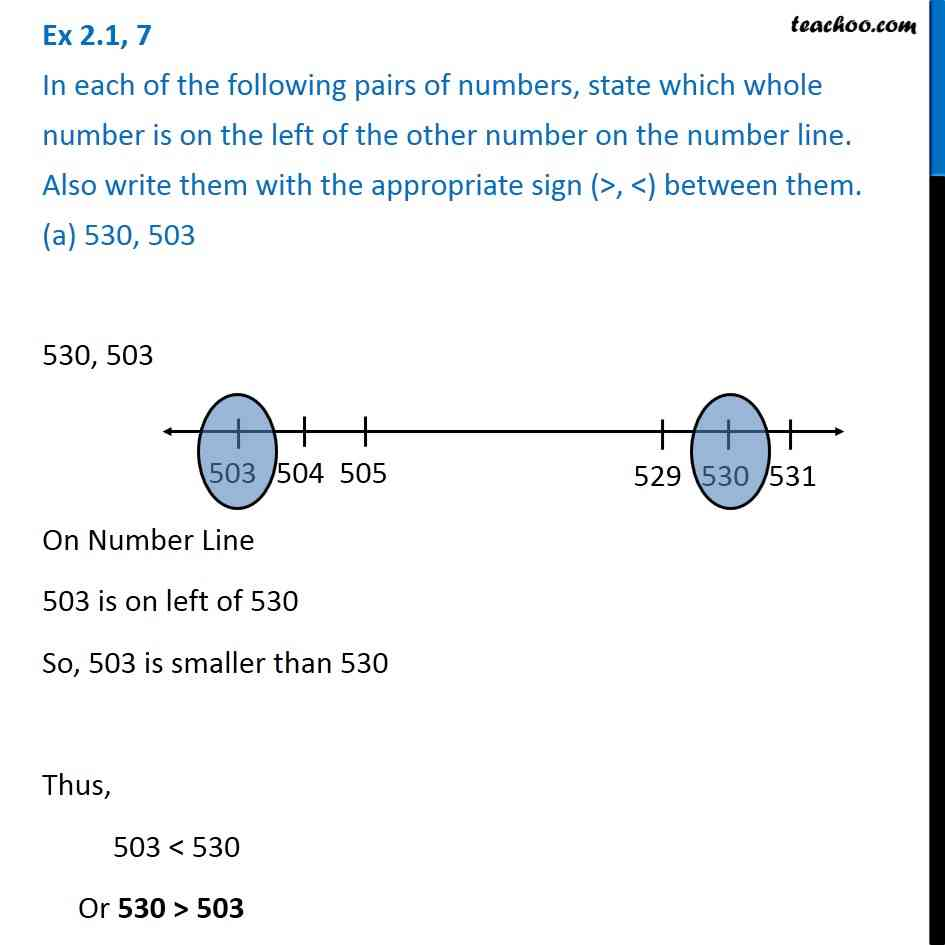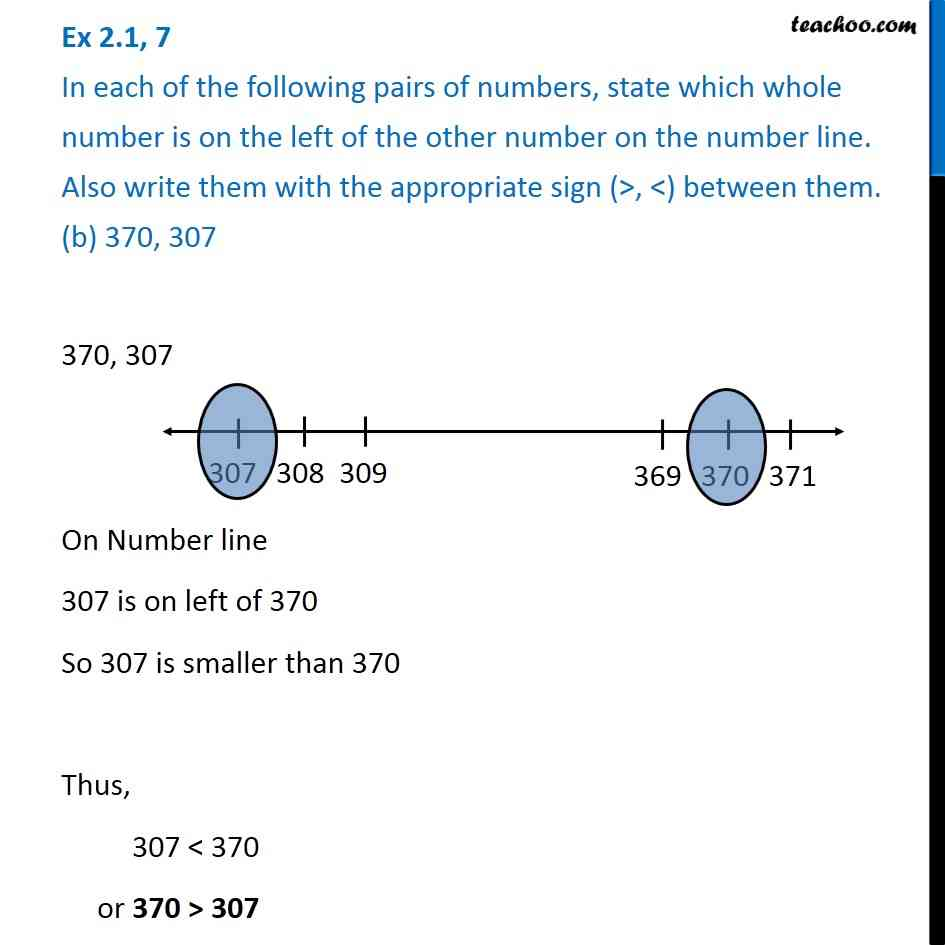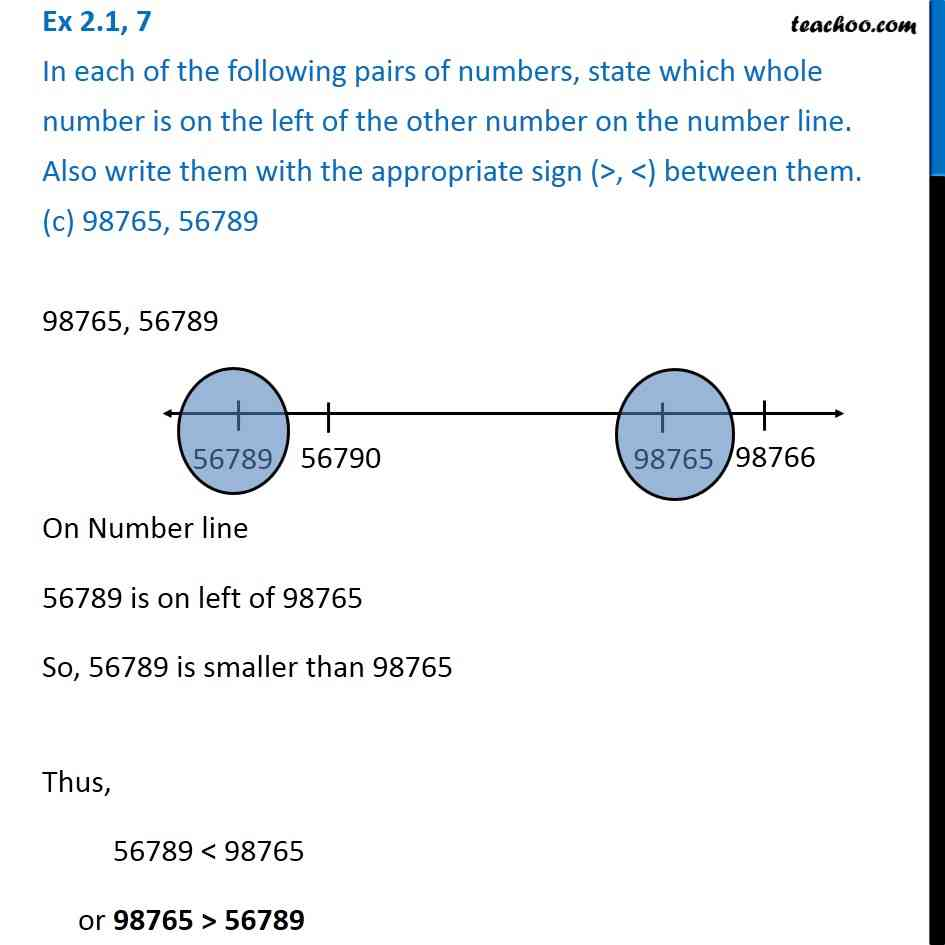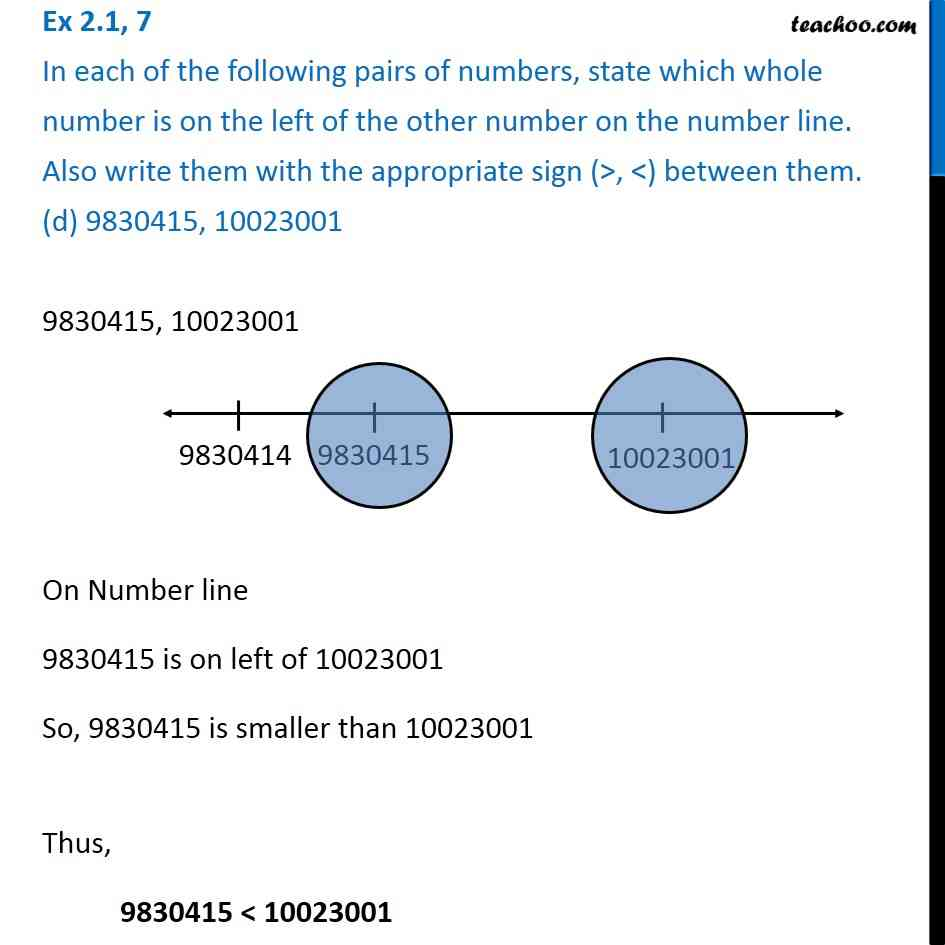Subscribe to our Youtube Channel - https://you.tube/teachoo

1. Chapter 2 Class 6 Whole Numbers
2. Serial order wise
3. Ex 2.1

Transcript

Ex 2.1, 7 In each of the following pairs of numbers, state which whole number is on the left of the other number on the number line. Also write them with the appropriate sign (>, <) between them. (a) 530, 503 530, 503 On Number Line 503 is on left of 530 So, 503 is smaller than 530 Thus, 503 < 530 Or 530 > 503 Ex 2.1, 7 In each of the following pairs of numbers, state which whole number is on the left of the other number on the number line. Also write them with the appropriate sign (>, <) between them. (b) 370, 307 370, 307 On Number line 307 is on left of 370 So 307 is smaller than 370 Thus, 307 < 370 or 370 > 307 Ex 2.1, 7 In each of the following pairs of numbers, state which whole number is on the left of the other number on the number line. Also write them with the appropriate sign (>, <) between them. (c) 98765, 56789 98765, 56789 On Number line 56789 is on left of 98765 So, 56789 is smaller than 98765 Thus, 56789 < 98765 or 98765 > 56789 Ex 2.1, 7 In each of the following pairs of numbers, state which whole number is on the left of the other number on the number line. Also write them with the appropriate sign (>, <) between them. (d) 9830415, 10023001 9830415, 10023001 On Number line 9830415 is on left of 10023001 So, 9830415 is smaller than 10023001 Thus, 9830415 < 10023001

Ex 2.1